# Objectives

charAt · compareTo · substring · objects · classes · object state · attributes · bahaviours · class design · constructors · Spot

# Strings - charAt()

Create a new Processing sketch in your workspace and call it Example_4_1.

## charAt() method

Include the following code in your sketch (don't cut and paste it, type it in):

``````String alphabet = "abcdefghijklmnopqrstuvwxyz";

println("The character at position 4 in "
+ alphabet
+ " is "
+ alphabet.charAt(3));

println("The character at position 10 in "
+ errorMessage404
+ " is "
+ errorMessage404.charAt(9));``````

Run your code; You should get the following output: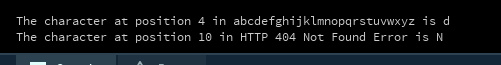# Strings - substring()

Create a new Processing sketch in your workspace and call it Example_4_2.

## substring() method

Type in the following code in your sketch:

``````//Version 1 (with roar variable)
String anotherPalindrome = "Niagara. O roar again!";
String roar = anotherPalindrome.substring(11, 15);
print(roar);``````

Run the code. Your output should be

``roar``

Now comment out the above code and add this code to your sketch:

``````//Version 2 (without roar variable)
String anotherPalindrome = "Niagara. O roar again!";
print(anotherPalindrome.substring(11, 15));

//Version 1 (with roar variable)
//String anotherPalindrome = "Niagara. O roar again!";
//String roar = anotherPalindrome.substring(11, 15);
//print(roar);``````

Run the code. Your output should still be:

``roar``

Do you understand the difference between the two code versions? In the first version, we declare a variable called 'roar'. In the second version, we don't use a 'roar' variable at all.

# Strings - compareTo()

We will step through a few examples of the compareTo method from your lectures.

## compareTo(), Example 4.3

Create a new Processing sketch in your workspace and call it Example_4_3.

Type in the following code in your sketch:

``````String str1 = "Dog";
String str2 = "Cat";

if(str1.compareTo(str2) < 0){
println(str1+" comes before "+ str2 +" in the alphabet");
}
else if(str1.compareTo(str2) > 0){
println(str2 +" comes before "+ str1+" in the alphabet");
}
else{
println("The strings are identical");
}``````

When you run it, you get the following output

``Cat comes before Dog in the alphabet``

Can you explain the output?

## compareTo(), Example 4.4

Then change the values of the two strings to the following:

``````String str1 = "cat";
String str2 = "Cat";``````

When you run it, you get the following output

``Cat comes before cat in the alphabet``

Can you explain the output?

## compareTo(), Example 4.5

Then change the values of the two strings to the following:

``````String str1 = "Animal";
String str2 = "Cat";``````

When you run it, you get the following output

``Animal comes before Cat in the alphabet``

Can you explain the output?

## compareTo(), Example 4.6

Then change the values of the two strings to the following:

``````String str1 = "Cat";
String str2 = "Cat";``````

When you run it, you get the following output

``The strings are identical``

# Strings - equals()

We will step through a new example relating to the equals method.

## equals()

Create a new Processing sketch in your workspace and call it lab04a_equals.

Type in the following code in your sketch:

``````String str1 = "a";
String str2 = "b";

if(str1.equals(str2))
{
println(str1+" is the same as "+ str2);
}
else
{
println(str1+" is NOT same as "+ str2);
}``````

Run this code and your output should be:

``a is NOT same as b``

Now make the following change so that both str1 and str2 have the value a:

``````String str1 = "a";
String str2 = "a";``````

When you run the code, your output should now be:

``a is the same as a``

# Objects and Classes

## Drawing spot without classes

Create a new Sketchbook and save it as Spot_Version0.0.

Enter the following code:

``````float x = 33.0;
float y = 50.0;
float diameter = 30.0;

void setup(){
size (100,100);
noStroke();
}

void draw(){
background(0);
ellipse(x, y, diameter, diameter);
}``````

Run the code. You should see a black window window with a white circle drawn in it.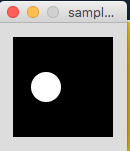## Drawing spot with basic class

Replace the existing code with this:

``````Spot sp;

void setup()
{
size (100,100);
noStroke();
sp = new Spot();
sp.xCoord = 33;
sp.yCoord = 50;
sp.diameter = 30;
}

void draw()
{
background(0);
ellipse(sp.xCoord, sp.yCoord, sp.diameter, sp.diameter);
}``````

Then using the new tab button as below, create a new tab called Spot. (This will be the name of the new class)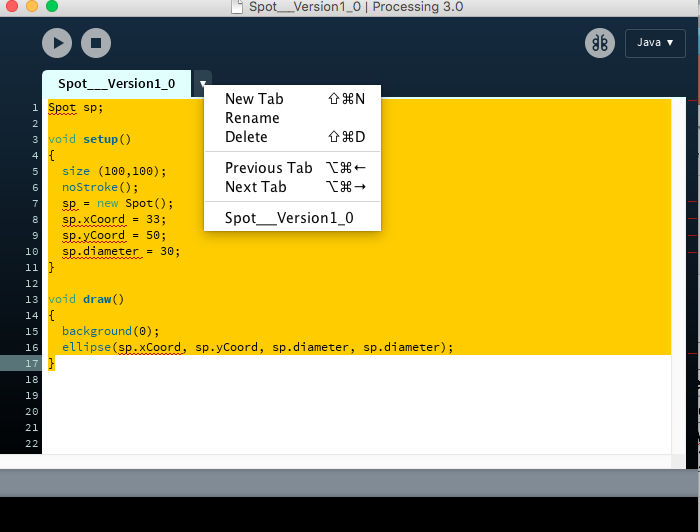Enter the following code in the new window.

``````class Spot
{
float xCoord, yCoord;
float diameter;
}``````

Run the code. You should see the same black window with a white circle drawn in it.## Drawing spot with Spot class and constructor

In the main tab, refactor the code so that it is the same as below:

``````Spot sp;

void setup()
{
size (100,100);
noStroke();
sp = new Spot(33, 50, 30);
}

void draw()
{
background(0);
ellipse(sp.xCoord, sp.yCoord, sp.diameter, sp.diameter);
}``````

Then go to the tab/class called Spot and refactor the code so that is has the following constructor:

``````class Spot
{
float xCoord, yCoord;
float diameter;

//Constructor
Spot(float xPos, float yPos, float diamtr)
{
xCoord = xPos;
yCoord = yPos;
diameter = diamtr;
}
}``````

Run the code. You should see the same black window with a white circle drawn in it.## Drawing spot with Spot class and multiple constructors

In this step, we will make no changes in the main tab.

Go to the tab/class called Spot and refactor the code so that is also has the default constructor:

``````class Spot{
float xCoord, yCoord;
float diameter;

//Default Constructor
Spot(){
}

//Constructor
Spot(float xPos, float yPos, float diamtr){
xCoord = xPos;
yCoord = yPos;
diameter = diamtr;
}
}``````

Run the code. Once again, you should see the same black window with a white circle drawn in it.# Exercise

In these exercises, we will look at solving some common errors.

## Exercise 1: Using ''='' in test for equality (syntax error)

Create a new sketch and save it as lab04a_exercise_1.

Cut and paste the following code into it:

``````        int num1 = 1;
int num2 = 2;

if(num1 = num2)
println(num1+" is the same as "+ num2);
else
println(num1+" is NOT same as "+ num2);``````

Try to run the code. You will see an error similar to this one: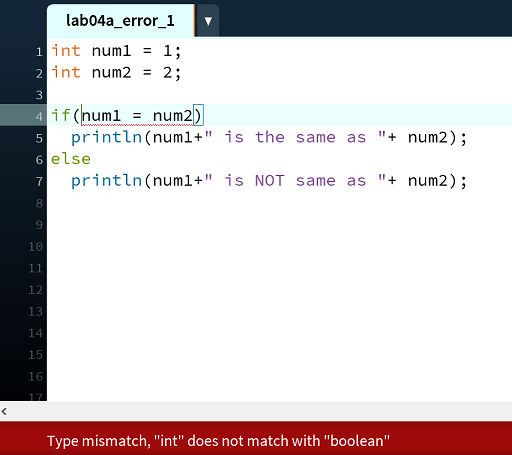Change the single ''='' to a double equals ''=='' Rerun the code. It should now work.

## Exercise 2: Using '';'' in incorrect place(logic)

Replace the code in it with this (cut and paste is fine here!):

``````int num1 = 1;
int num2 = 2;

if(num1 == num2) ;
println(num1+" is the same as "+ num2);
if (num1 != num2)
println(num1+" is NOT same as "+ num2);``````

Run the code. You will get the folowing (incorrect) output.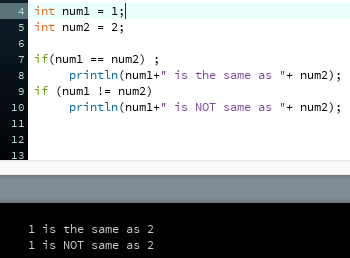Can you see where the error is? Change the code to that you get the correct output ("1 is not the same as 2").

If you are having difficulty spotting it, the error is in the first if statement...there should be no ; after the boolean expression.

## Exercise 3: Not using ''{'' and ''}'' to control statements(logic)

Replace the code in it with this (cut and paste is fine here!):

``````int num1 = 1;
int num2 = 2;

if(num1 == num2)
println(num1+" is the same as "+ num2);
println("This means that they are equal");
if (num1 != num2)
println(num1+" is NOT same as "+ num2);``````

Run the code. You will get the folowing (incorrect) output.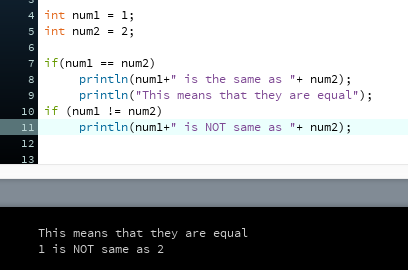Can you see where the error is? Hint: there is a problem with curly braces...recall that curly braces are not required in an if statement when there is only one statement in it; they are required when there is more than one statement.

Change the code to that you get the correct output in each case (when numbers are equal and when numbers are not equal).

# Challenges

In these challenges, we will bring in validation on our fields in the constructor. If you have difficulty attempting these, don't worry...we will cover this approach in detail in your next lecture!

## Challenge 1

Using Spot Version 3.0 as a starting point, save this as lab04a_challenge_01.

In this code, you will notice we have the following Spot Constructor:

``````Spot(float xPos, float yPos, float diamtr){
xCoord = xPos;
yCoord = yPos;
diameter = diamtr;
}``````

We want to add some validation rules to this i.e.

• before setting the diameter, check that the value sent into the constructor is between 20 and 50 inclusive. If the value sent in is outside that range, set a default value of 20.

• before setting the xPos and the yPos, check that the value sent into the constructor is not negative (i.e. >= 0). If the value sent in is negative, set a default value of 0.

Test your code to make sure it is behaving appropriately.

Save your work and close the project.

## Challenge 2

If we add another field to Spot - a String field called spotName. (This simply stores the name of the spot). The class now looks like (without the validations done above):

``````class Spot{
String spotName;
float xCoord, yCoord;
float diameter;

Spot(String name, float xPos, float yPos, float diamtr){
spotName = name;
xCoord = xPos;
yCoord = yPos;
diameter = diamtr;
}
}``````

and the main class looks like:

``````Spot sp;

void setup()
{
size (100,100);
noStroke();
sp = new Spot( "SpottySpot", 33, 50, 30);
}

void draw()
{
background(0);
ellipse(sp.xCoord, sp.yCoord, sp.diameter, sp.diameter);
println(sp.spotName);
}``````

Create a new sketch called lab04a_challenge_02 and cut and paste the above code into it.

Firstly, run this program. See how the code in the Spot class and the main class have changed to cater for the extra field. Can you explain why so many SpottySpot are printed to the console?

Then, add a validation to the constructor that only allows names that are no more than 7 characters long. If the name is too long, the constructor should impose the rule by cutting the spotName string to the first 7 characters.

Test your code to make sure it is behaving appropriately. In particular, perform the following tests:

• enter a name that is 6 characters long...all 6 characters should be stored in the name.

• enter a name that is 7 characters long...all 6 characters should be stored in the name.

• enter a name that is 8 characters long...only the first 7 characters should be stored in the name (hint: substring).

NOTE: This is called boundary testing i.e. we are testing just below, exactly on, and just above the boolean condition that we used in the validation. This is a popular testing approach as a lot of bugs live in the boundaries.

Save your work and close the project.

# Solutions

The solutions for this lab can be found here.

If you require unzipping software, 7zip is a good choice: http://www.7-zip.org/ (or Keka for Mac http://www.kekaosx.com/en/)

After unzipping the solutions, it might be a good idea to copy the folders to your sketchbook folder. From Processing, you could then use File, followed by Sketchbook to easily open them.